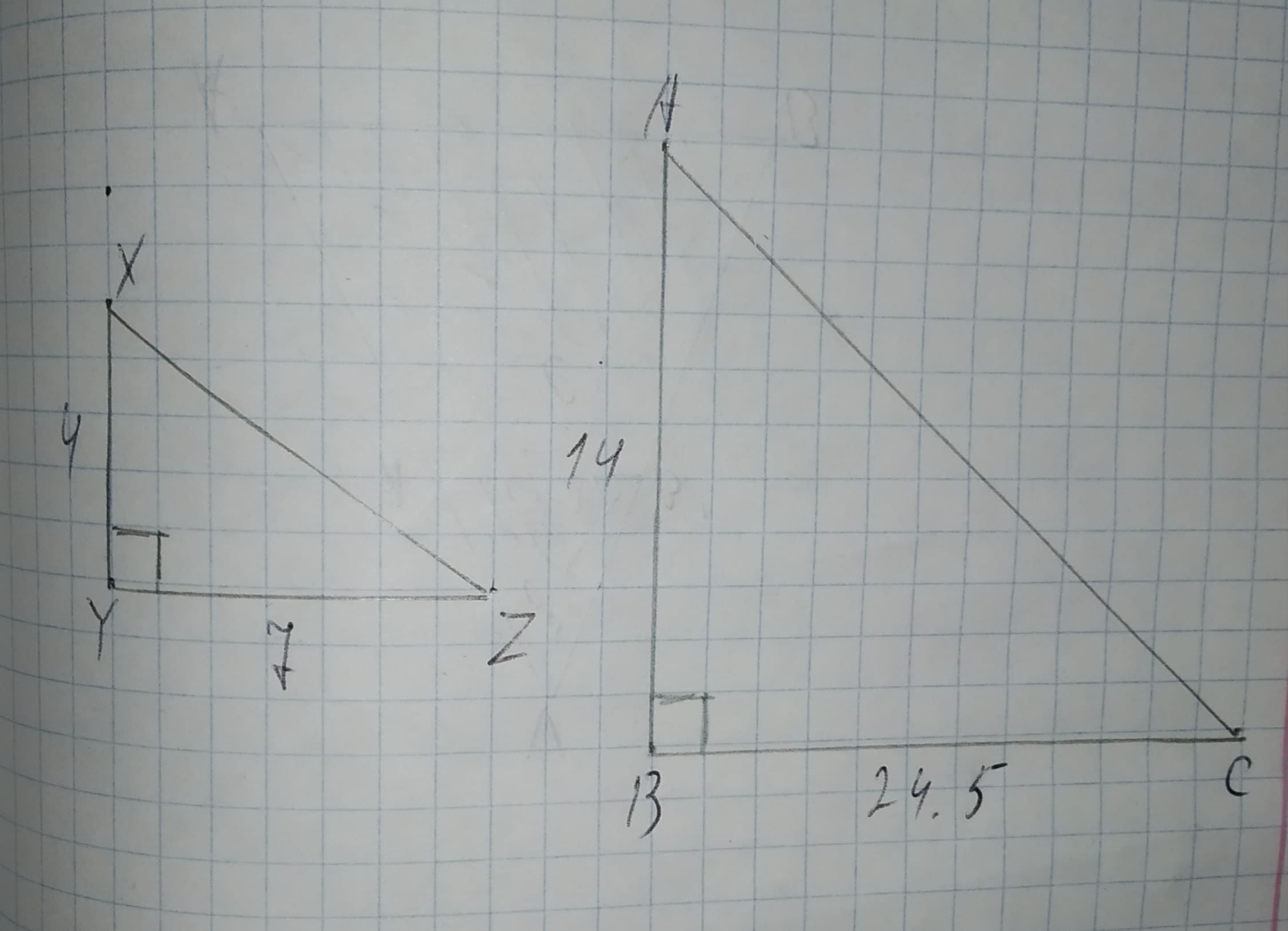Question# To determine:Whether each pair of figures is similar and also write similarity statement and scale factor.Given information:Given the figure.12210203002.jpg

Similarity
ANSWEREDTo determine:Whether each pair of figures is similar and also write similarity statement and scale factor.
Given information:
Given the figure.2020-12-07

Calculation:
When the lenght of the triangles are proportional to the lenght of two corresponding sides of another triangle and the included angles are congruent.
So, the triangles are similar by SAS similarity theorem.
Given that $$\displaystyle\angle{Y}{\quad\text{and}\quad}\angle{B}$$ are right angles.
Therefore,
$$\displaystyle\angle{Y}\stackrel{\sim}{=}\angle{B}$$The ratio of corresponding sides
$$\displaystyle\frac{{{X}{Y}}}{{{A}{B}}}=\frac{{4}}{{14}}$$
$$\displaystyle=\frac{{2}}{{7}}$$
And,
$$\displaystyle\frac{{{Y}{Z}}}{{{B}{C}}}=\frac{{7}}{{24.5}}$$
$$\displaystyle=\frac{{2}}{{7}}$$
So, $$\displaystyle\frac{{{X}{Y}}}{{{A}{B}}}=\frac{{{Y}{Z}}}{{{B}{C}}}=\frac{{2}}{{7}}$$
Therefore, $$\displaystyle\triangle{X}{Y}{Z}\sim\triangle{A}{B}{C}$$ by SAS similarity theorem and the scale factor is $$\displaystyle\frac{{2}}{{7}}$$.